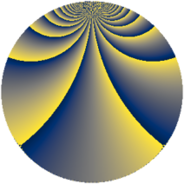# Properties

 Label 2736.3.wLevel $2736$ Weight $3$ Character orbit 2736.w Rep. character $\chi_{2736}(37,\cdot)$ Character field $\Q(\zeta_{4})$ Dimension $796$ Sturm bound $1440$

# Related objects

## Defining parameters

 Level: $$N$$ $$=$$ $$2736 = 2^{4} \cdot 3^{2} \cdot 19$$ Weight: $$k$$ $$=$$ $$3$$ Character orbit: $$[\chi]$$ $$=$$ 2736.w (of order $$4$$ and degree $$2$$) Character conductor: $$\operatorname{cond}(\chi)$$ $$=$$ $$304$$ Character field: $$\Q(i)$$ Sturm bound: $$1440$$

## Dimensions

The following table gives the dimensions of various subspaces of $$M_{3}(2736, [\chi])$$.

Total New Old
Modular forms 1936 804 1132
Cusp forms 1904 796 1108
Eisenstein series 32 8 24

## Trace form

 $$796q - 4q^{4} + 4q^{5} + O(q^{10})$$ $$796q - 4q^{4} + 4q^{5} + 4q^{11} + 52q^{16} + 8q^{17} - 34q^{19} - 80q^{20} + 56q^{26} + 72q^{38} - 4q^{43} - 268q^{44} + 8q^{47} - 5412q^{49} + 80q^{58} + 60q^{61} + 396q^{62} + 224q^{64} - 440q^{68} + 432q^{74} + 4q^{76} - 192q^{77} + 408q^{80} + 300q^{82} + 164q^{83} - 424q^{85} - 192q^{92} + 388q^{95} + O(q^{100})$$

## Decomposition of $$S_{3}^{\mathrm{new}}(2736, [\chi])$$ into newform subspaces

The newforms in this space have not yet been added to the LMFDB.

## Decomposition of $$S_{3}^{\mathrm{old}}(2736, [\chi])$$ into lower level spaces

$$S_{3}^{\mathrm{old}}(2736, [\chi]) \cong$$ $$S_{3}^{\mathrm{new}}(304, [\chi])$$$$^{\oplus 3}$$$$\oplus$$$$S_{3}^{\mathrm{new}}(912, [\chi])$$$$^{\oplus 2}$$